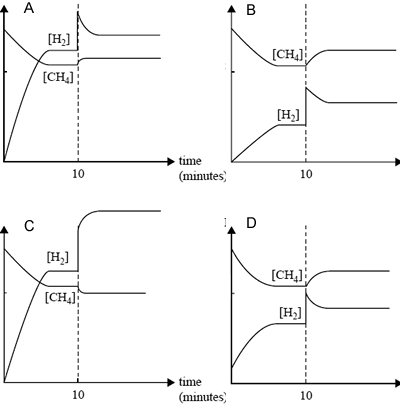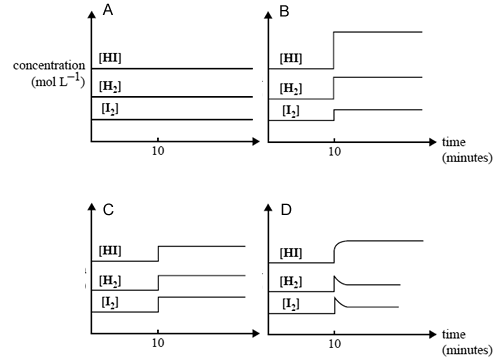Equilibrium chemistry 2008 VCE The following gaseous equilibrium is established at high temperatures in the presence of a finely divided nickel (Ni) catalyst. CH4(g) + H2O(g) <=> CO(g) + 3H2(g); ΔH = +206 kJ mol–1 A particular reaction is carried out using equal amounts of CH4(g) and H2O(g). Which one of the following sets of changes in conditions would lead to the greatest increase in the proportion of the reactants converted to products? A. Volume of reaction vessel increased, temperature increased B. Volume of reaction vessel increased, temperature decreased C. Volume of reaction vessel decreased, temperature increased D. Volume of reaction vessel decreased, temperature decreased SolutionThis reaction mentioned above occurs at a measurable rate only when the finely divided catalyst is present. This catalyst increases the reaction rate because A. it strongly attracts the reaction products, driving the reaction to the right. B. the reactants can become attached to its surface where they can meet and undergo reaction. C. it provides energy to the reactants when their molecules bounce off it, increasing the proportion of molecules in the gas state with the required activation energy. D. it increases the equilibrium constant of the reaction, causing an increase in the proportion of products at equilibrium. SolutionEqual amounts of CH4(g) and H2O(g) are added to a reaction vessel and allowed to react. After 10 minutes, equilibrium has been reached. At that time, some H2 is added to the mixture and equilibrium is reestablished. Which one of the following graphs, mol/L vs time, best represents the changes in the amounts of CH4 and H2 in the reaction mixture?A B C D SolutionHydrogen iodide dissociates into its elements according to the following equation. 2HI(g) <=> H2(g) + I2(g) ΔH = +9 kJ mol–1 A mixture of H2(g), I2(g) and HI(g) rapidly comes to equilibrium in a 2.0 L container. After the reaction has been at equilibrium for 10 minutes, the volume of the container is suddenly reduced to 1.3 L at constant temperature. Which one of the following graphs best represents the effect of this decrease in volume on the concentration of the gases in the mixture?A B C D SolutionPhosphorus (V) chloride, PCl5, decomposes to form phosphorus (III) chloride, PCl3, and chlorine, Cl2 according to the equation PCl5(g) <=> PCl3(g) + Cl2(g) Four different fl asks, A, B, C and D, at the same temperature, contain a mixture of PCl5, PCl3 and Cl2. The concentration, in mol L–1, of these components in each of the flasks is shown below. In three of the four flasks, the mixture of gases is at equilibrium. In which one is the mixture of gases not at equilibrium? Flask [PCl5(g)] [PCl3(g)] [Cl2(g)] A. [PCl5(g)] = 0.15, [PCl3(g)] = 0.20, [Cl2(g)] = 0.30 B. [PCl5(g)] = 0.20, [PCl3(g)] = 0.15, [Cl2(g)] = 0.15 C. [PCl5(g)] = 0.10, [PCl3(g)] = 0.10, [Cl2(g)] = 0.40 D. [PCl5(g)] = 0.30, [PCl3(g)] = 0.80, [Cl2(g)] = 0.15 Solution Some gaseous PCl5 is placed in an empty container. When equilibrium is reached, the mass of the gas mixture, compared to the initial mass of PCl5, is A. halved. B. unchanged. C. one and a half times greater. D. doubled. SolutionGaseous NOCl decomposes to form the gases NO and Cl2 according to the following equation. 2NOCl(g) <=>2NO(g) + Cl2(g) The numerical value of the equilibrium constant for this reaction is 1.6 × 10–5 at 35°C. What is the numerical value of the equilibrium constant, at 35°C., for the following reaction? NO(g) + 1/2 Cl2(g) <=> NOCl(g) A. –1.6 × 10–5 B. 1.6 × 10–5 C. 2.5 × 102 D. 6.3 × 104 SolutionDinitrogen tetroxide, N2O4(g), dissociates to form nitrogen dioxide, NO2(g), according to the equation N2O4(g) <=>2NO2(g) 0.45 mol of N2O4 gas is placed in an empty 1.00 L vessel at 100°C. When the system reaches equilibrium, there is 0.36 mol of NO2 gas present in the vessel. Calculate the numerical value of the equilibrium constant for this reaction at 100°C. Solution At 25°C, the numerical value of the equilibrium constant for this reaction is 0.144. Is this reaction endothermic or exothermic? Give an explanation for your answer. Solution